# Programme for International Student Assessment (PISA) 2018: highlights from Scotland's results

Report covering Scotland's performance in the Programme for International Student Assessment (PISA) 2018, covering reading, maths and science.

### 5. Performance in Mathematics

5.1 Scotland's performance in Mathematics

1. Scotland's mean score in Mathematics in 2018 was 489. This was similar to what it was in 2015 (491), 2012 (498) and 2009 (499) but lower than in 2006 (506) and 2003 (524). See Chart 5.1.1 for more information.

2. Scotland's standard deviation in Mathematics in 2018 was 95 points. This was higher than in 2015 (84 points), 2012 (86 points) and 2006 (85 points but similar to 2009 (93 points).

3. In 2018 in Scotland, performance among boys was higher than among girls in Mathematics (497 vs 481). This was also the case in 2006, 2009, 2012, but not in 2015 where their performance was similar (Chart 5.1.2 and Chart 5.1.3).

4. In 2018, the performance of second generation immigrant students (512), first generation immigrant students (500) and non-immigrant students (490) was similar.

5. In 2018, 23.5 per cent of students in Scotland performed below PISA Level 2 in Mathematics. This was similar to 2009 and 2015, but higher than 2006 and 2012. In 2018, 10.6 per cent of students in Scotland performed at PISA Level 5 or better in Mathematics. This was similar to 2006, 2009, 2012 and 2015 (Chart 5.1.4).

6. In 2018, 13.0 per cent of boys performed at PISA Level 5 or better in Mathematics, which was higher than the proportion of girls (8.3 per cent). In 2018, the proportion of students performing below PISA Level 2 in Mathematics was similar (25.2 per cent of girls and 21.7 per cent of boys).

7. The share of variation in Mathematics test scores that was explained by students' background was 7.9 per cent. This was similar to 2015 and 2012 but lower than 2006 (14.6 per cent) and 2009 (16.3 per cent).

8. The ESCS gradient shows how much score varies on average with each step (one point) in social background. The ESCS gradient was 31 points in the mathematics assessment for Scotland. This was similar to 2006 (41 points), 2012 (37 points) and 2015 (33 points), but lower than in 2009 (45.5 points).

9. The difference between the 5th and 95th percentiles by ESCS was 2.72 points. Combined with a 31-point gradient, this implies that their average scores in mathematics are apart by 83 points which implies a difference of just under three years' schooling.

Chart 5.1.1 Scotland's PISA mathematics scores, 2003-2018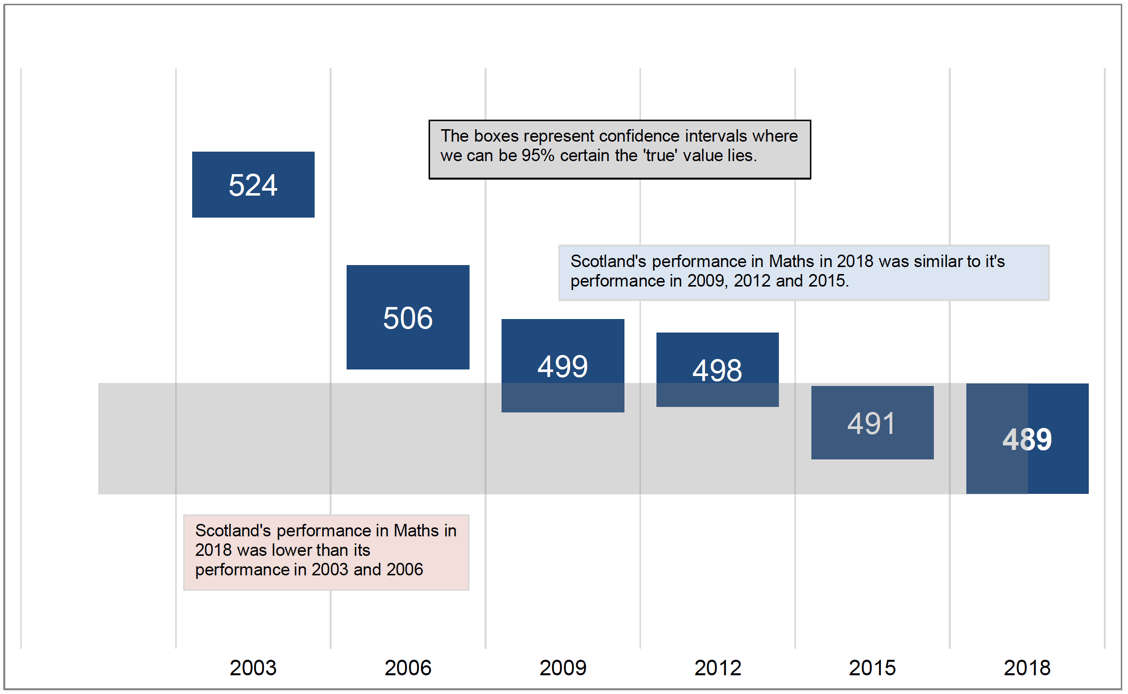Chart 5.1.2 Scotland's PISA mathematics scores among girls, 2006-2018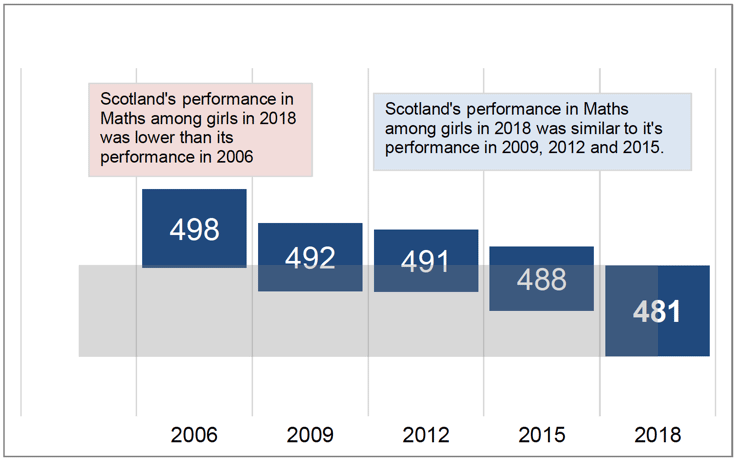Chart 5.1.3 Scotland's PISA mathematics scores among boys, 2006-2018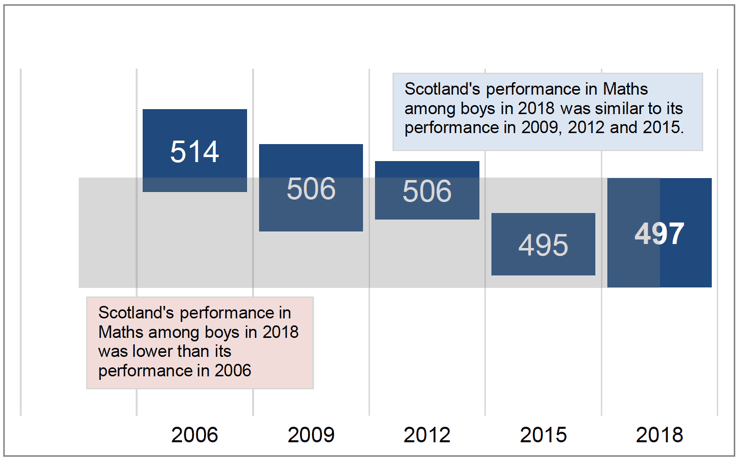Chart 5.1.4 Scotland's mathematics scores, by PISA Proficiency Level, 2006-2018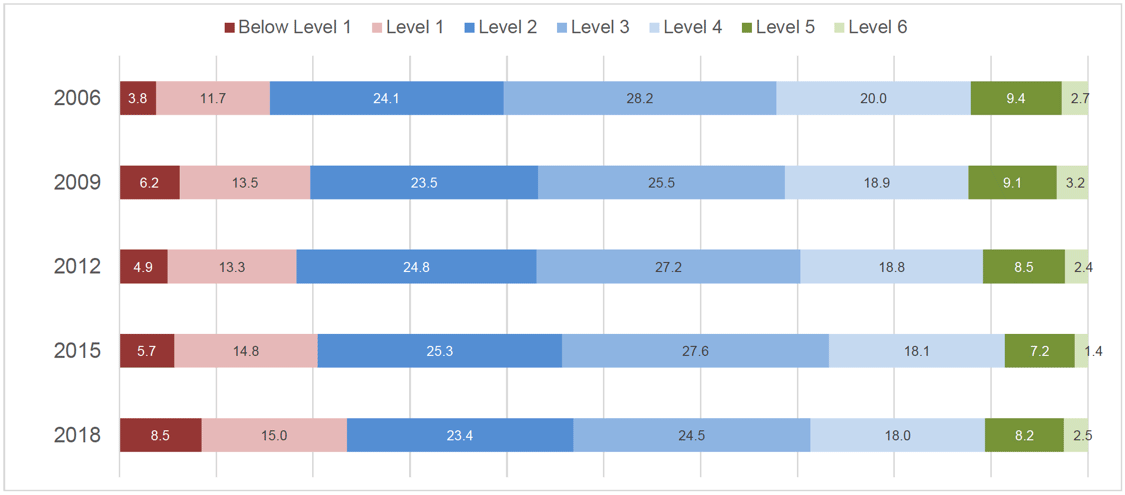As set out in Chapter 2, the OECD categorise students into Levels according to their ability to undertake certain tasks. However the group below Level 2 merits particular attention, as the OECD consider that Level 2 is the baseline of ability to participate effectively in society.

In 2018, 23.5% of students in Scotland performed below PISA Level 2 in Mathematics. This was similar to 2009 and 2015, but higher than 2006 and 2012. In 2018, 10.6% of students in Scotland performed at PISA Level 5 or better in Mathematics. This was similar to 2006, 2009, 2012 and 2015.

5.2 Scotland's performance in Mathematics relative to countries in the OECD and UK administrations

1. Scotland's mean score in Mathematics in 2018 of 489 was higher than seven countries, including the United States (478). It was similar to 14 countries, including Northern Ireland (492), Wales (487) and the OECD average (489). It was lower than 18 countries, including England (504) and the UK as a whole (502). See Chart 5.2.1 for more information. Comparisons to non-OECD countries can be found on our new PISA dashboard.

2. Scotland's mean score in Mathematics among girls in 2018 was higher than six countries, similar to 12 countries and the OECD average and lower than 21 countries and the UK as a whole (Chart 5.2.2).

3. Scotland's mean score in Mathematics among boys in 2018 was higher than nine countries, similar to 19 countries, the OECD average and the UK as a whole and lower than 11 countries (Chart 5.2.3).

4. Scotland's gender gap in Mathematics of -16 points (girls – boys) was lower than nine countries, similar to 30 countries, the OECD average and the UK as a whole and higher than no countries.

5. In 2018, second generation immigrant students in Scotland (512) performed higher than or similar to all OECD countries in Mathematics, with only Singapore and Macao (China) of the non-OECD countries having a higher performance than Scotland. Performance among first generation immigrant students in Scotland (500) was also higher than or similar to all OECD countries in Mathematics.

6. In 2018, 23.5 per cent of students in Scotland performed below PISA Level 2 in Mathematics. This was higher than 16 countries and also higher than the UK as a whole. It was similar to 16 countries and the OECD average and lower than seven countries (Chart 5.2.4).

7. 10.6 per cent of students in Scotland performed at PISA Level 5 or better in Mathematics. This was lower than 10 countries, similar to 21 countries (plus the UK and the OECD average) and higher than eight countries (Chart 5.2.5).

8. The share of variation in mathematics test scores that was explained by students' background was 7.9 per cent (Chart 5.2.6). Among OECD countries and UK administrations, this was lower than 18 countries, including the OECD average (13.8 per cent), similar to 21 countries and higher than no countries.

9. The ESCS gradient shows how much score varies on average with each step (one point) in social background (Chart 5.2.7). The ESCS gradient was 31 points in the mathematics assessment for Scotland. Among OECD countries and UK administations, this was lower than eight countries, similar to 31 countries and higher than no countries.

Chart 5.2.1 PISA mathematics scores of OECD countries (plus three other UK administrations), relative to Scotland, 2018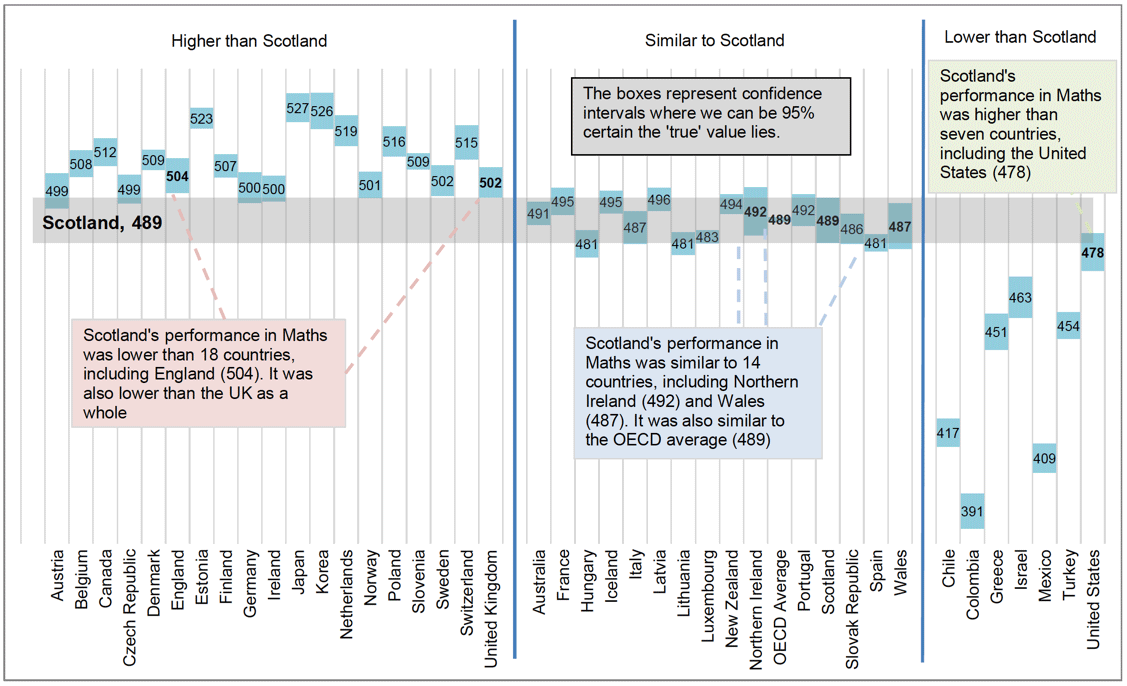Chart 5.2.2 PISA mathematics scores among girls in OECD countries, relative to Scotland, 2018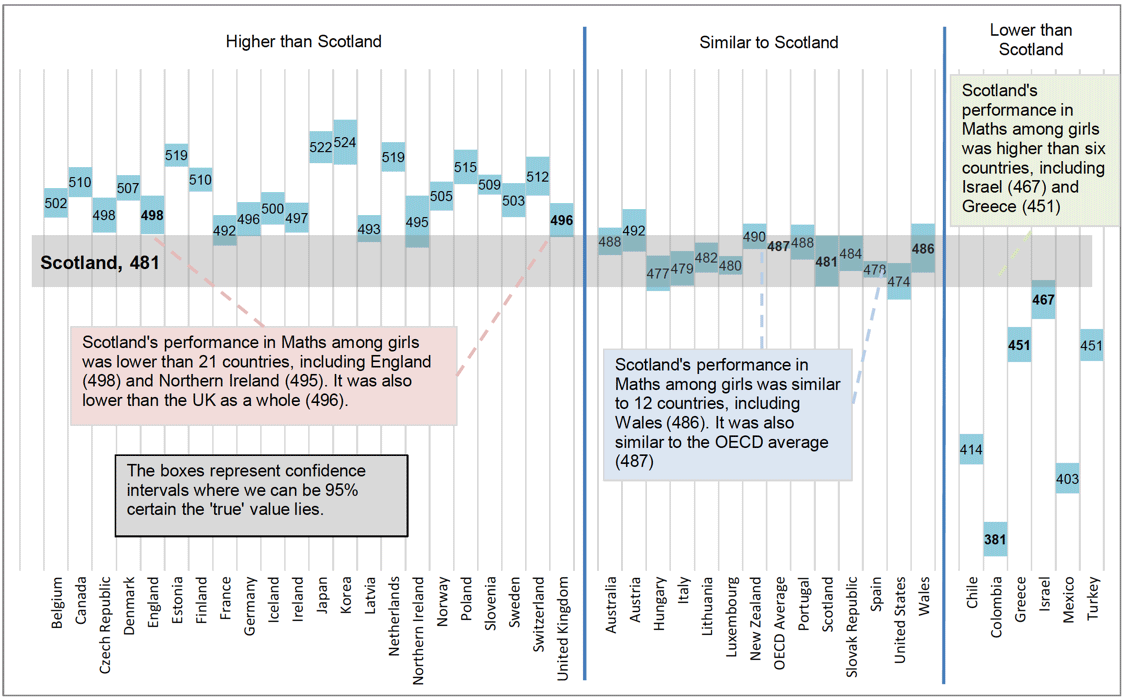Chart 5.2.3 PISA mathematics scores among boys in OECD countries, relative to Scotland, 2018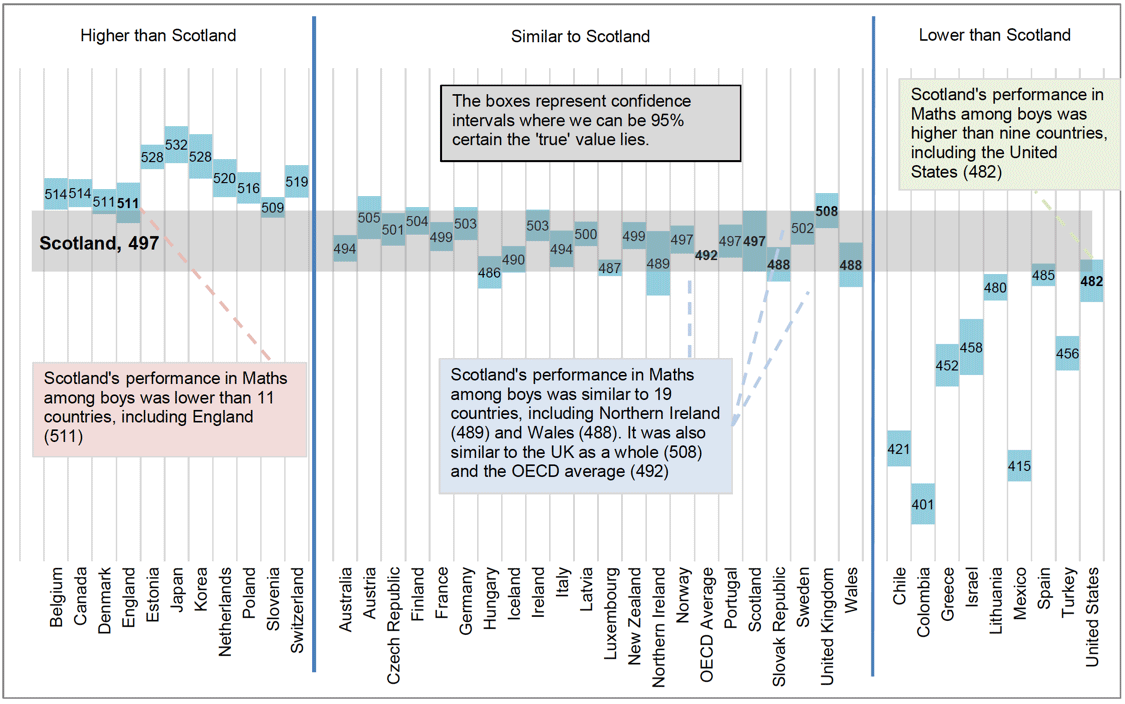Chart 5.2.4 Proficiency Levels in Mathematics in OECD countries, arranged by percentage of students below Level 2, 2018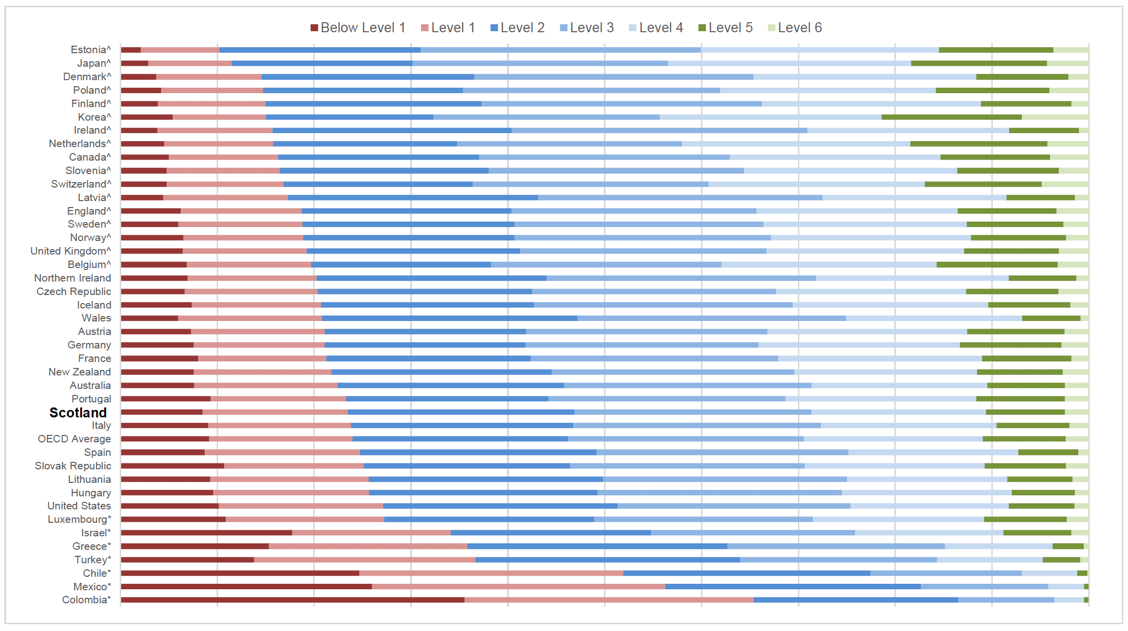In 2018, 23.5% of students in Scotland performed below PISA level 2 in Mathematics. This was higher (^) than 16 countries and the United Kingdom as a whole, similar to 16 countries and the OECD average and lower (*) than seven countries.

Chart 5.2.5 Proficiency Levels in Mathematics in OECD countries, by percentage of students at Level 5 or better, 2018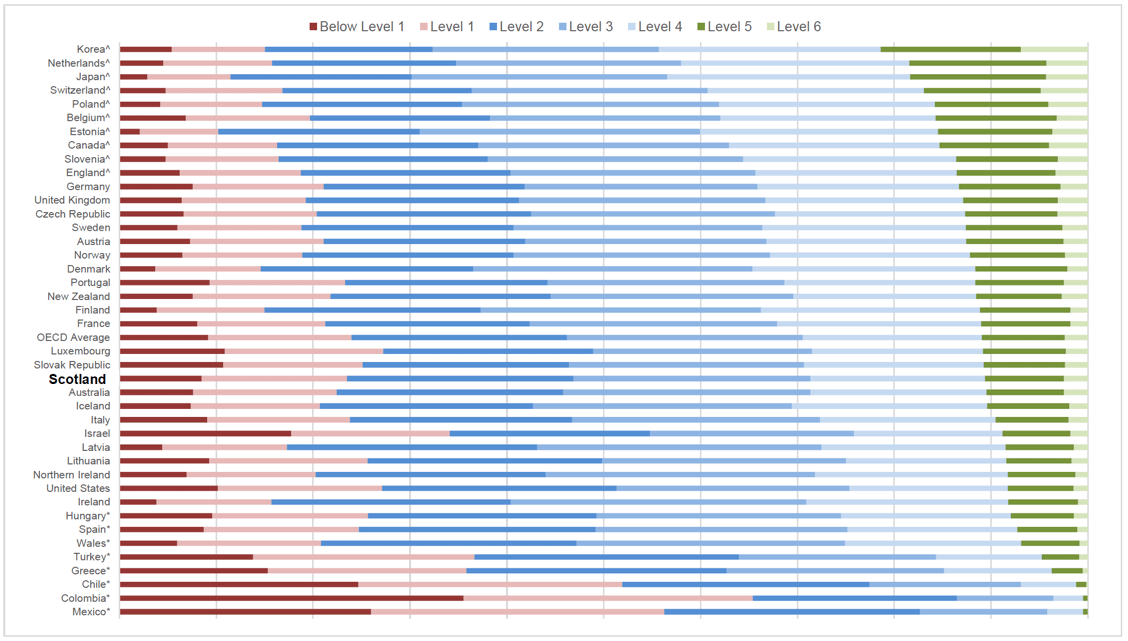In 2018, 10.6% of students in Scotland performed at PISA Level 5 or better in Mathematics. This was higher (*) than eight countries, similar to 21 countries, the UK and the OECD average and lower (^) than 10 countries.

Chart 5.2.6 Share of variation in Mathematics performance explained by ESCS in OECD countries, relative to Scotland, 2018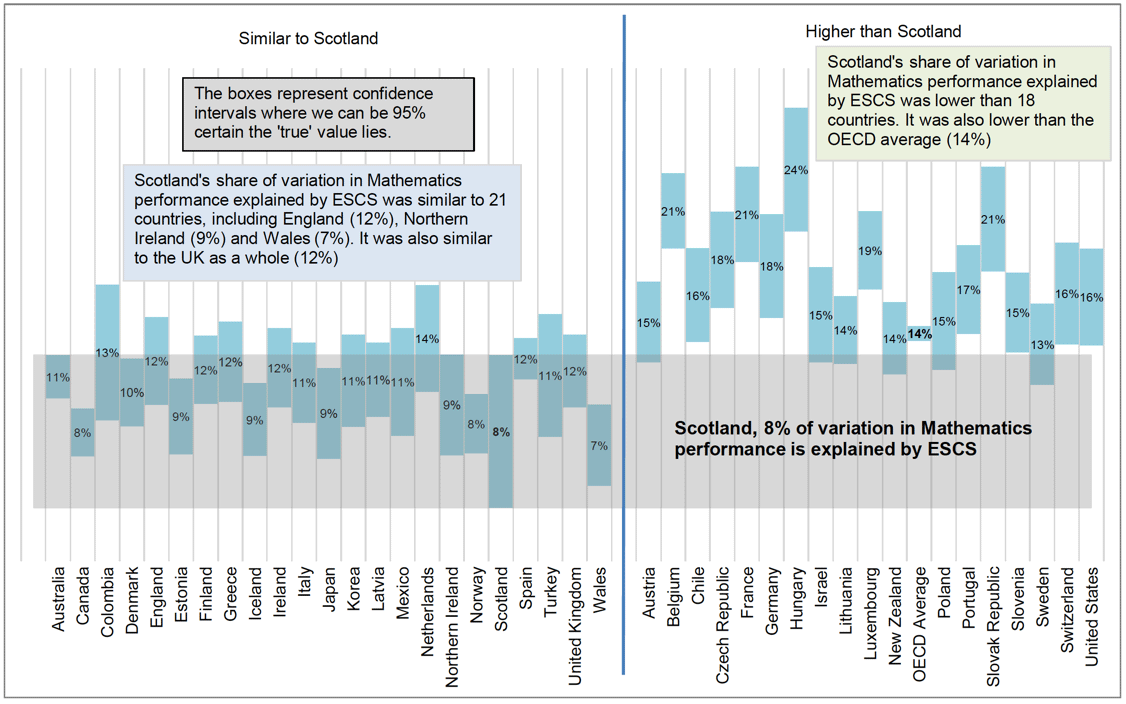Chart 5.2.7 ESCS gradient in Mathematics in OECD countries, relative to Scotland, 2018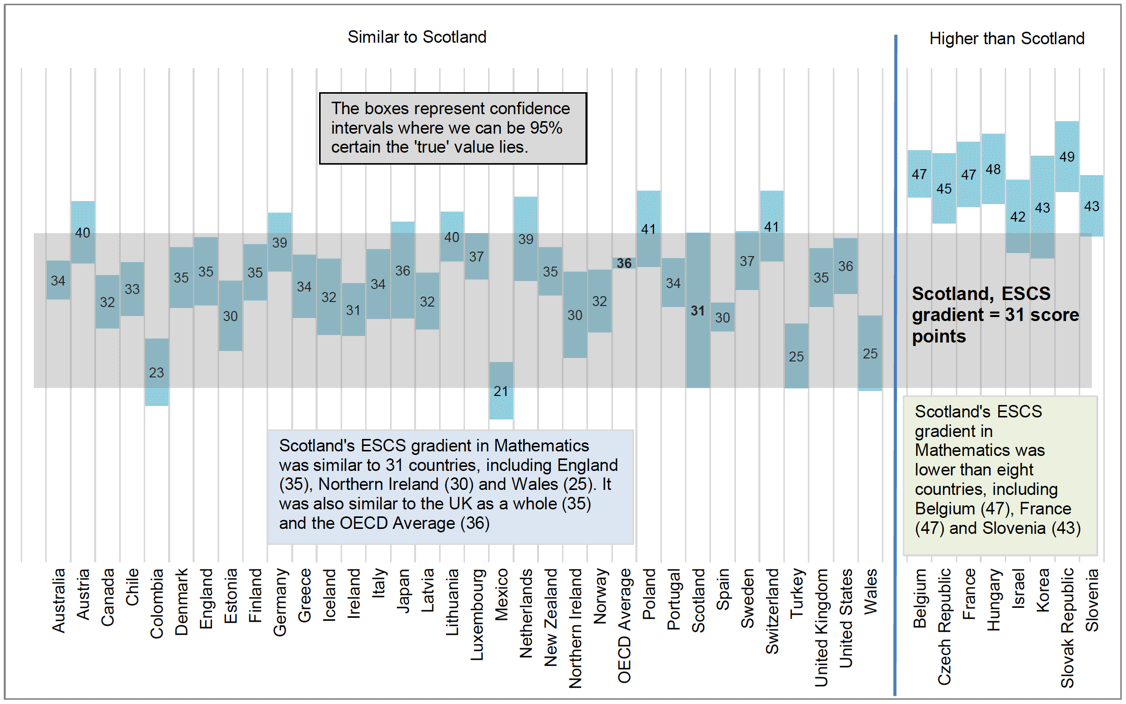### Contact

Email: keith.dryburgh@gov.scot